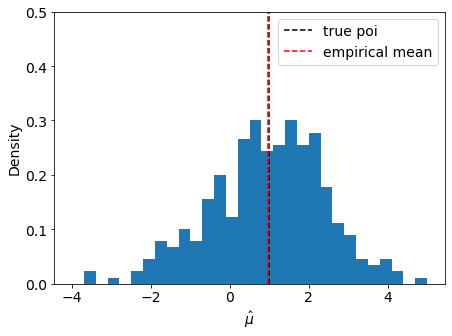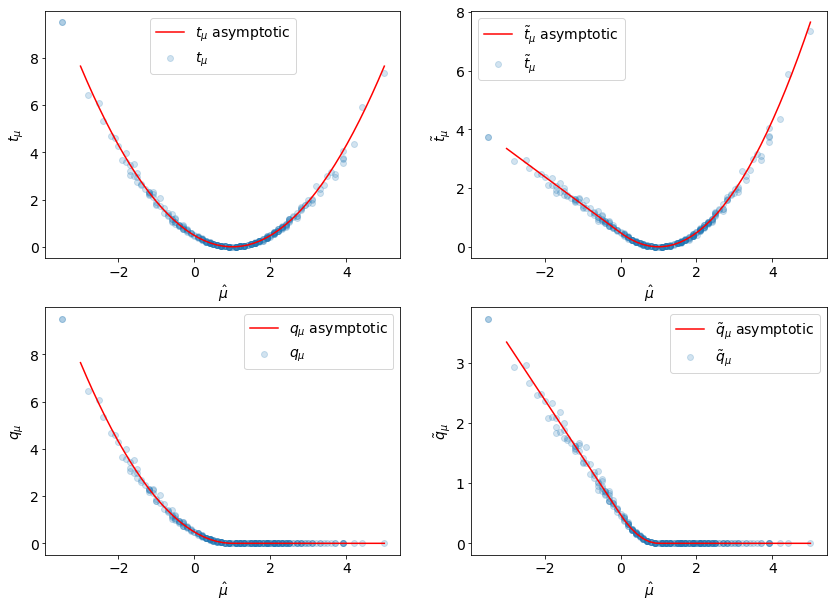# Empirical Test Statistics¶

In this notebook we will compute test statistics empirically from pseudo-experiment and establish that they behave as assumed in the asymptotic approximation.

:

import numpy as np
import matplotlib.pyplot as plt
import pyhf

np.random.seed(0)
plt.rcParams.update({"font.size": 14})


First, we define the statistical model we will study.

• the signal expected event rate is 10 events

• the background expected event rate is 100 events

• a 10% uncertainty is assigned to the background

The expected event rates are chosen to lie comfortably in the asymptotic regime.

:

model = pyhf.simplemodels.hepdata_like(
signal_data=[10.0], bkg_data=[100.0], bkg_uncerts=[10.0]
)


The test statistics based on the profile likelihood described in arXiv:1007.1727 cover scanarios for both

• POI allowed to float to negative values (unbounded; $$\mu \in [-10, 10]$$)

• POI constrained to non-negative values (bounded; $$\mu \in [0,10]$$)

For consistency, test statistics ($$t_\mu, q_\mu$$) associated with bounded POIs are usually denoted with a tilde ($$\tilde{t}_\mu, \tilde{q}_\mu$$).

We set up the bounds for the fit as follows

:

unbounded_bounds = model.config.suggested_bounds()
unbounded_bounds[model.config.poi_index] = (-10, 10)

bounded_bounds = model.config.suggested_bounds()


Next we draw some synthetic datasets (also referrerd to as “toys” or pseudo-experiments). We will “throw” 300 toys:

:

true_poi = 1.0
n_toys = 300
toys = model.make_pdf(pyhf.tensorlib.astensor([true_poi, 1.0])).sample((n_toys,))


In the asymptotic treatment the test statistics are described as a function of the data’s best-fit POI value $$\hat\mu$$.

So let’s run some fits so we can plots the empirical test statistics against $$\hat\mu$$ to observed the emergence of the asymptotic behavior.

:

pars = np.asarray(
[pyhf.infer.mle.fit(toy, model, par_bounds=unbounded_bounds) for toy in toys]
)


We can now calculate all four test statistics described in arXiv:1007.1727

:

test_poi = 1.0
tmu = np.asarray(
[
pyhf.infer.test_statistics.tmu(
test_poi,
toy,
model,
init_pars=model.config.suggested_init(),
par_bounds=unbounded_bounds,
)
for toy in toys
]
)

:

tmu_tilde = np.asarray(
[
pyhf.infer.test_statistics.tmu_tilde(
test_poi,
toy,
model,
init_pars=model.config.suggested_init(),
par_bounds=bounded_bounds,
)
for toy in toys
]
)

:

qmu = np.asarray(
[
pyhf.infer.test_statistics.qmu(
test_poi,
toy,
model,
init_pars=model.config.suggested_init(),
par_bounds=unbounded_bounds,
)
for toy in toys
]
)

:

qmu_tilde = np.asarray(
[
pyhf.infer.test_statistics.qmu_tilde(
test_poi,
toy,
model,
init_pars=model.config.suggested_init(),
par_bounds=bounded_bounds,
)
for toy in toys
]
)


Let’s plot all the test statistics we have computed

:

muhat = pars[:, model.config.poi_index]
muhat_sigma = np.std(muhat)


We can check the asymptotic assumption that $$\hat{\mu}$$ is distributed normally around it’s true value $$\mu' = 1$$

:

fig, ax = plt.subplots()
fig.set_size_inches(7, 5)

ax.set_xlabel("$\hat{\mu}$")
ax.set_ylabel("Density")
ax.set_ylim(top=0.5)

ax.hist(muhat, bins=np.linspace(-4, 5, 31), density=True)
ax.axvline(true_poi, label="true poi", color="black", linestyle="dashed")
ax.axvline(np.mean(muhat), label="empirical mean", color="red", linestyle="dashed")
ax.legend();Here we define the asymptotic profile likelihood test statistics:

$t_\mu = \frac{(\mu-\hat\mu)^2}{\sigma^2}$
$\begin{split}\tilde{t}_\mu = \begin{cases} t_\mu,\;\text{\hat{\mu}>0}\\ t_\mu - t_0,\; \text{else} \end{cases}\end{split}$
$\begin{split}q_\mu = \begin{cases} t_\mu,\;\text{\hat{\mu}<\mu}\\ 0,\; \text{else} \end{cases}\end{split}$
$\begin{split}\tilde{q}_\mu = \begin{cases} \tilde{t}_\mu,\;\text{\hat{\mu}<\mu}\\ 0,\; \text{else} \end{cases}\end{split}$
:

def tmu_asymp(mutest, muhat, sigma):
return (mutest - muhat) ** 2 / sigma ** 2

def tmu_tilde_asymp(mutest, muhat, sigma):
a = tmu_asymp(mutest, muhat, sigma)
b = tmu_asymp(mutest, muhat, sigma) - tmu_asymp(0.0, muhat, sigma)
return np.where(muhat > 0, a, b)

def qmu_asymp(mutest, muhat, sigma):
return np.where(
muhat < mutest, tmu_asymp(mutest, muhat, sigma), np.zeros_like(muhat)
)

def qmu_tilde_asymp(mutest, muhat, sigma):
return np.where(
muhat < mutest, tmu_tilde_asymp(mutest, muhat, sigma), np.zeros_like(muhat)
)


And now we can compare them to the empirical values:

:

muhat_asymp = np.linspace(-3, 5)
fig, axarr = plt.subplots(2, 2)
fig.set_size_inches(14, 10)

labels = ["$t_{\mu}$", "$\\tilde{t}_{\mu}$", "$q_{\mu}$", "$\\tilde{q}_{\mu}$"]
data = [
(tmu, tmu_asymp),
(tmu_tilde, tmu_tilde_asymp),
(qmu, qmu_asymp),
(qmu_tilde, qmu_tilde_asymp),
]

for ax, (label, d) in zip(axarr.ravel(), zip(labels, data)):
empirical, asymp_func = d
ax.scatter(muhat, empirical, alpha=0.2, label=label)
ax.plot(
muhat_asymp,
asymp_func(1.0, muhat_asymp, muhat_sigma),
label=f"{label} asymptotic",
c="r",
)
ax.set_xlabel(r"$\hat{\mu}$")
ax.set_ylabel(f"{label}")
ax.legend(loc="best")